# Convert Excel Date to Unix Time Stamp (or Time Stamp to Excel Date)

This post will guide you how to convert a date string to a Unix time stamp in Excel. How do I convert excel date to Unix timestamp with a formula in Excel. How to convert Unix time stamp to an excel date string in Excel.

## Convert Excel Date to Unix Time Stamp

If you want to convert the excel date string to unix time stamp in Excel, you can create a formula based on the DATE function.

For Example, you have a list of data that contain dates, and you want to convert each date to unix time stamp. You can use the following formula.

`=(A1-DATE(1970,1,1))*86400`

Type this formula into a blank cell and press Enter key and then drag the AutoFill handle from Cell B1 to B5.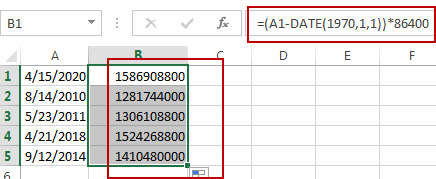## Convert Unix Time Stamp to Excel Date

To convert a unix time stamp to an excel date string in Excel, you can use a formula based on the Date function. Like this:

`=(((B1/60)/60)/24)+DATE(1970,1,1)`

Type this formula into a cell and press Enter key, and then drag the AutoFill Handle from Cell C1 to C5.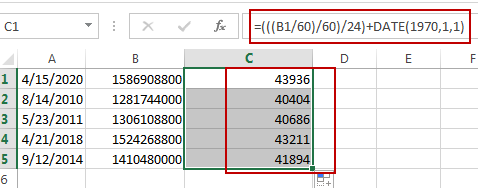Then you need to select the range of cells C1:C5, and right click on it, and select Format Cell from the popup menu list. And the Format Cell dialog will open. Click Date under Category list box, and then click OK button.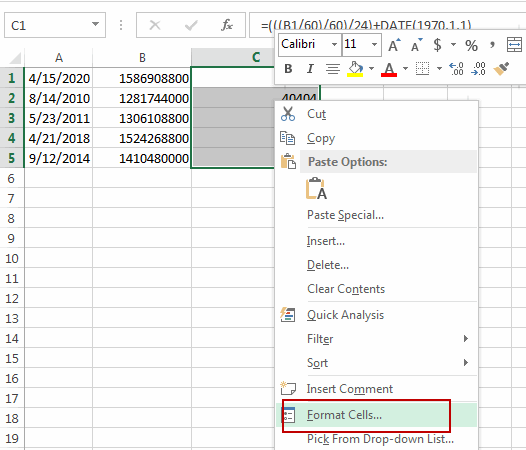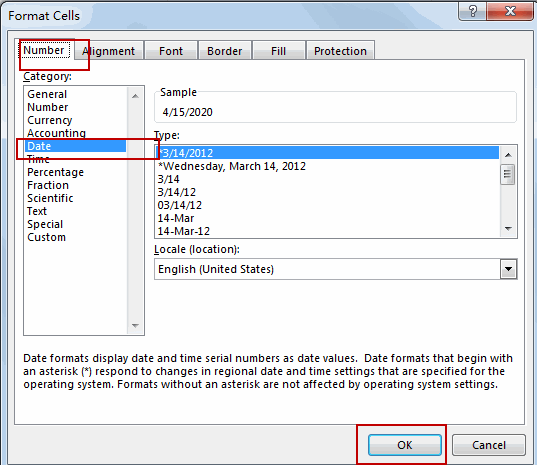Let’s see the result: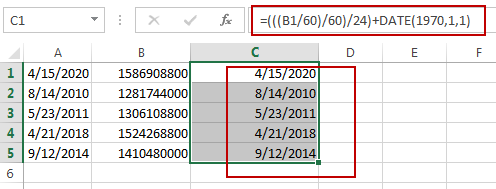### Related Functions

• Excel DATE function
The Excel DATE function returns the serial number for a date.The syntax of the DATE function is as below:= DATE (year, month, day)…